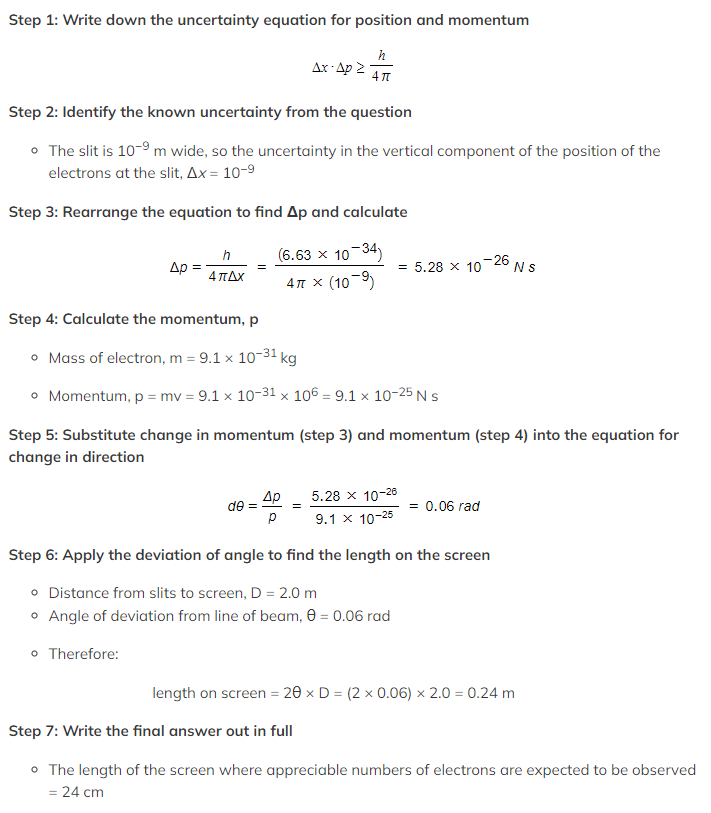# IB DP Physics: HL复习笔记12.1.9 The Uncertainty Principle

### The Uncertainty Principle

• The quantisation of energy levels and their associated wave functions leads to some surprising outcomes
• One of these outcomes is known as the uncertainty principle
• The uncertainty principle states that:
There are certain pairs of physical quantities that are cannot be known precisely at the same time
• This leads to two consequences related to:
• Position and momentum
• Energy and time

#### Position & Momentum

• One example is that it is not possible to know the position and the momentum of a quantum particle precisely
• This can be represented in equation form as:
•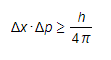Where:
• Δx = change in displacement
• Δpx = change in momentum
• h = Planck's constant
• This equation shows that:
• The better the position of the particle is known, the less precise is the knowledge of its momentum
• The value of Planck’s constant is small so this limitation only becomes important for small particles within the quantum regime - it does not apply in the Newtonian mechanics that you have used to calculate momentum for larger objects

#### Energy & Time

• There is a similar relationship between energy and time where both cannot be known precisely
•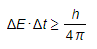Where:
• ΔE = change in energy
• Δt = change in time
• h = Planck's constant

#### Wavefunctions

• This principle can be demonstrated by looking at the wavefunctions associated with quantum particles that are nearly 'free'
• For example, those associated with the energy levels in a 'swimming pool' potential energy well
• The graph of wavefunction vs position shows that while the momentum is known precisely the position of the particle is unknown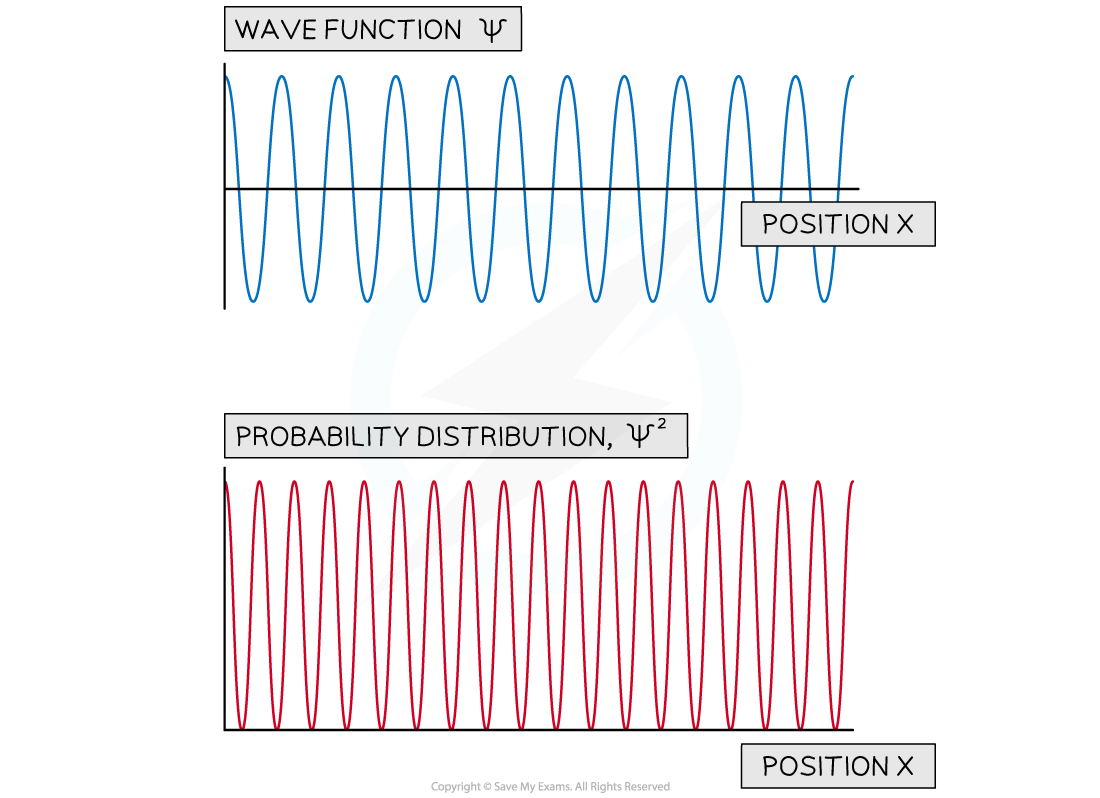The wave function of a free particle with a fixed energy. The wave function shows it has a single value of momentum while the probability distributions shows that the particle can be anywhere in space.

• For a particle in a 'swimming pool' potential well, its energy levels are close together
• Hence, the wave function can be a mixture of the wavefunctions associated with a number of closely spaced energy levels
• For a range of five neighbouring energy levels, the wavefunction can be obtained by adding the individual wavefunctions
• This is a result of the principle of superposition
• This gives rise to a wave packet, meaning the particle is more likely to be in a particular region of space
• This can be shown by a graph of the probability distribution vs position
• This helps to visualise how the approximate uncertainty in the position, Δx and momentum Δpx can be determinedA wave packet made from the wavefunctions associated with five closely spaced energy levels. The probability distribution is shown along with the uncertainty in position and momentum.

• Consider the wavefunctions associated with five and ten energy levels with the probability distribution and the estimates of Δx and Δpx
• Comparing the two sets of graphs, it can be seen that:
• As the momentum becomes less well known the position becomes more well known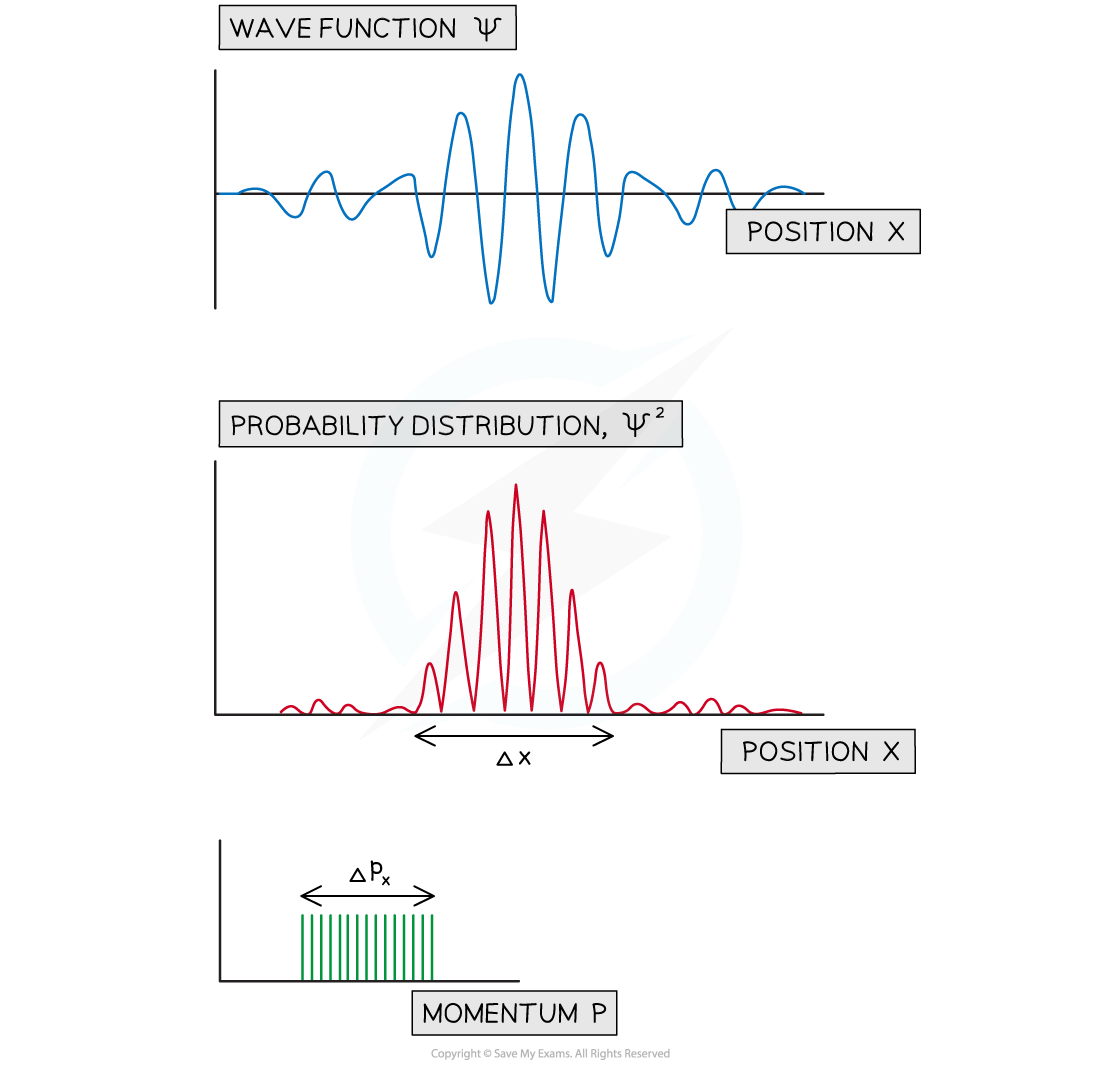A wave packet made from the wavefunctions associated with five closely spaced energy levels. The probability distribution is shown along with the uncertainty in position and momentum.

#### Worked Example

A narrow beam of electrons with velocity 106 m s−1 are directed towards a slit which is 10-9 m wide.

The electrons are observed on a screen placed 2.0 m from the slit.

Estimate the length of the area of the screen where electrons will be seen in appreciable numbers.